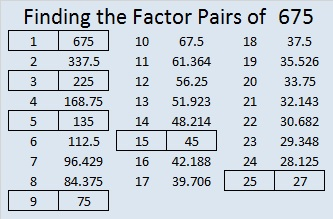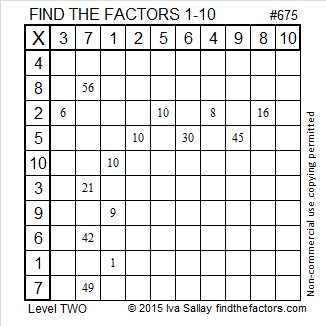# 675 and Level 2

675 is made from 3 consecutive numbers so 675 is divisible by 3. Since the middle number of those consecutive numbers, 6, is divisible by 3, we know that 675 is also divisible by 9.

Since the last two digits of 675 is a multiple of 25, we know that 675 is divisible by 25.

Since 5 and 25 are two of its factors, 675 is the hypotenuse of two Pythagorean triples: 405-540-675 and 189-648-675. What is the greatest common factor of each set of three numbers?

The numbers in one of 675’s factor pairs, 25 and 27, are each exactly one number away from 26, their average. That means we are just one number away from 26².

Thus, 675 equals 26² – 1² which can be factored into (26 + 1)(26 – 1) so (26 + 1)(26 – 1) = 27 x 25 = 675.Print the puzzles or type the solution on this excel file: 10 Factors 2015-11-09

—————————————————————————————————

• 675 is a composite number.
• Prime factorization: 675 = 3 x 3 x 3 x 5 x 5, which can be written 675 = (3^3) x (5^2)
• The exponents in the prime factorization are 3 and 2. Adding one to each and multiplying we get (3 + 1)(2 + 1) = 4 x 3 = 12. Therefore 675 has exactly 12 factors.
• Factors of 675: 1, 3, 5, 9, 15, 25, 27, 45, 75, 135, 225, 675
• Factor pairs: 675 = 1 x 675, 3 x 225, 5 x 135, 9 x 75, 15 x 45, or 25 x 27
• Taking the factor pair with the largest square number factor, we get √675 = (√225)(√3) = 15√3 ≈ 25.98076.—————————————————————————————————